Harmonic measure

A concept in the theory of harmonic functions (cf. Harmonic function) connected with estimating the modulus of an analytic function inside a domain if certain bounds on the modulus on the boundary of the domain are known , . Letbe a bounded open set in the Euclidean space,; letbe the boundary of; and letbe a finite real-valued continuous function on. To each such functionthere corresponds a unique harmonic functiononwhich, for, is a generalized solution of the Dirichlet problem. If the point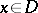is assumed to be fixed, the functionalwill define on the compact seta positive Radon measure, which is called the harmonic measure at the point. The formula for the representation of the generalized solution of the Dirichlet problem,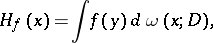obtained by Ch.J. de la Vallée-Poussin by the balayage method, is valid for all functionswhich are continuous on. Moreover, ifis an arbitrary Borel set on, the harmonic measure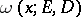,, ofatis equal to the value atof the generalized solution of the Dirichlet problem for the characteristic function,, of.

The basic properties of a harmonic measure are:is a harmonic function of the pointin;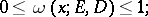ifis a domain andeven at a single point, then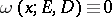.

In the last-named caseis known as a set of harmonic measure zero. If a compact set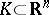has harmonic measure zero with respect to some domain,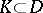, i.e.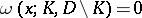, then it has harmonic measure zero with respect to all other domains, i.e.is a set of absolute harmonic measure zero. A sethas absolute harmonic measure zero if and only if it has zero (harmonic) capacity.

As regards applications to the theory of functions of a complex variable, the dependence of a harmonic measure on the domainis of special importance. This dependence is expressed by the principle of harmonic measure (cf. Harmonic measure, principle of), which states that a harmonic measure does not decrease under mappings of the domainrealized by univalent analytic functions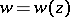,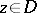. In particular, a harmonic measure remains unchanged under a one-to-one conformal mapping.

Explicit computations of harmonic measures are possible only for the simplest domains(mainly for the disc, for the sphere, for a half-plane, and for a half-space; see Poisson integral). This is the reason for the importance of the various estimation methods for harmonic measure , , , , which are mainly based on the principle of extension of domain (cf. Extension of domain, principle of). In the simplest form, for, this principle consists in the following: Let a finitely-connected domainbe bounded by a finite number of Jordan curvesand letbe an arc on. Then, if the domainis extended in some way across the complementary part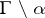of the boundary, the harmonic measure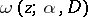can only increase.